RS Aggarwal Class 8 Solutions Chapter 17 - Construction Of Quadrilaterals

RS Aggarwal Class 8 Chapter 17 - Construction Of Quadrilaterals Solutions Free PDF

RS Aggarwal class 8 solutions chapter 17 construction of quadrilaterals is provided here. Solving the RS Aggarwal questions will help the students to understand the concepts in a better and comprehensive way. After solving the questions from this chapter the students will learn the properties of quadrilaterals like the diagonals of a quadrilateral are congruent, opposite sides are parallel to each other and many more.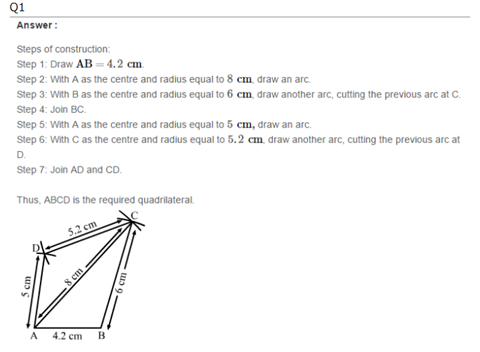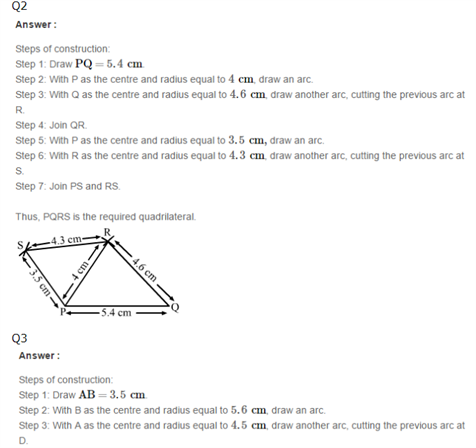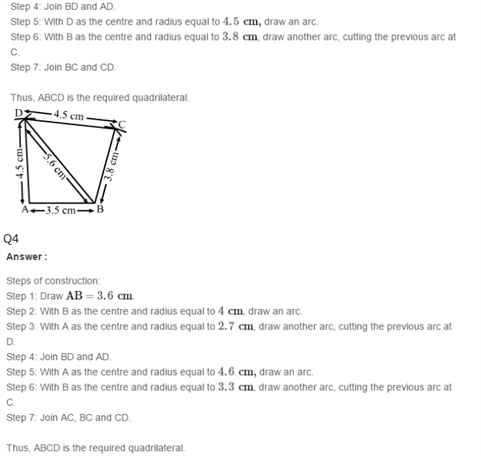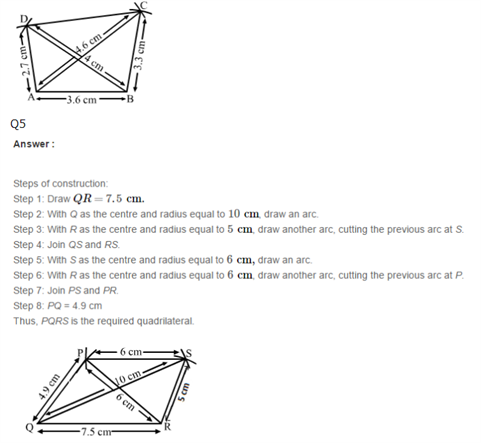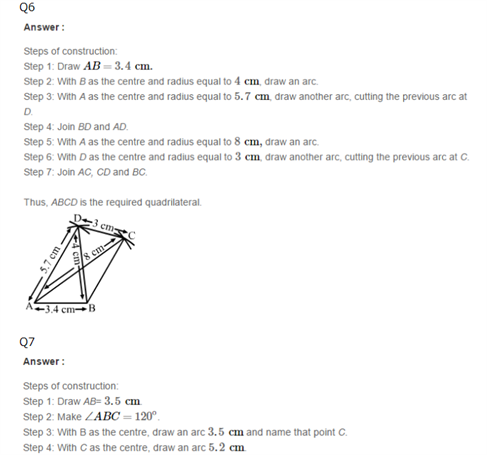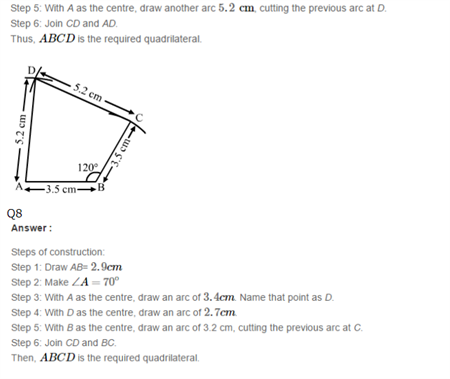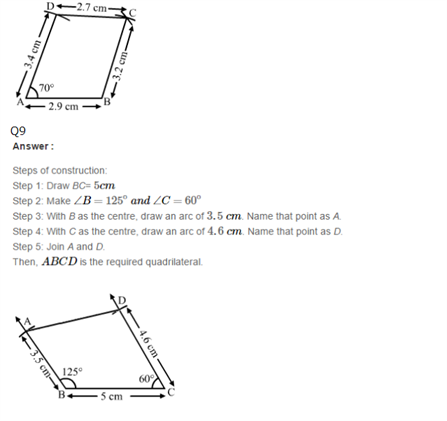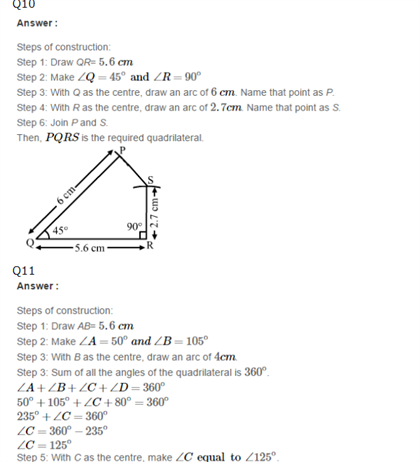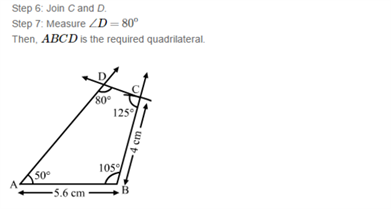81